# Civil Engineering - Irrigation

### Exercise :: Irrigation - Section 1

41.

The discharge over a trapezoidal crest of a Sarda fall with free over fall conditions, is

 A. 0.45 2g . LH3/2 (H/B)1/3 B. 0.45 2g . LH2/3 (H/B)2/3 C. 0.45 2g . LH3/2 (H/B)1/6 D. 0.45 2g . LH1/6 (H/B)3/2

Explanation:

No answer description available for this question. Let us discuss.

42.

If q is the discharge per unit width of a channel and D1 D2 are the depths of water before and after hydraulic jump, the following relationship is true

 A.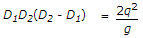B.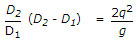C.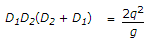D.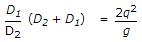Explanation:

No answer description available for this question. Let us discuss.

43.

A river training work is generally required when the river is

Explanation:

No answer description available for this question. Let us discuss.

44.

For loss of head in a canal inverted syphon barrel, the factor in the Unwin formula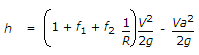is a coefficient for loss of head due to

 A. friction B. exit C. entry D. gradient

Explanation:

No answer description available for this question. Let us discuss.

45.

Pick up the correct statement from the following:

 A. If the flexibility is more than one, the outlet is hyper-proportional B. If the setting of an outlet is higher than that required for proportionality, the outlet is hyper-proportional C. If the setting of an outlet is lower than that required for proportionality, the outlet is sub-proportional D. If the flexibility is zero, it is a rigid module E. All the above.